• +91 9971497814
• info@interviewmaterial.com

# RD Chapter 20- Surface Areas and Volume of A Right Circular Cone Ex-VSAQS Interview Questions Answers

### Related Subjects

Question 1 : The height of a cone is 15 cm. If its volume is 500π cm3, then find the radius of its base.

Answer 1 : Volume of cone = 500π cm3
and height (h) = 15 cm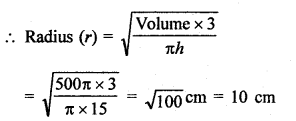Question 2 : If the volume of a right circular cone of height 9 cm is 48π cm3, find the diameter of its base.

Answer 2 : Volume of a cone = 48π cm3
Height (h) = 9 cm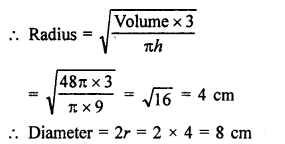Question 3 : If the height and slant height of a cone are 21 cm and 28 cm respectively. Find its volume.

Height of a cone (h) = 21 cm
and slant height (l) = 28 cm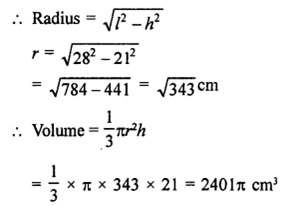Question 4 : The height of a conical vessel is 3.5 cm. If its capacity is 3.3 litres of milk. Find the diameter of its base.

Answer 4 : Capacity of conical vessel = 3.3 litres
Volume = 3.3 m3
= 3.3 x 1000 = 3300 cm2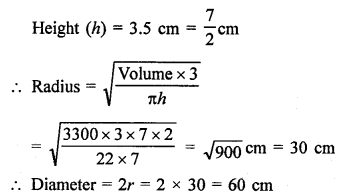Question 5 : If the radius and slant height of a cone are in the ratio 7 : 13 and its curved surface area is 286 cm2, find its radius.

Answer 5 : Two ratio in radius and slant height of a cone = 7 : 13
and slant height (1) = 13x
Curved surface area = πrl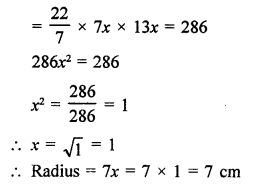Question 6 : Find the area of canvas required for a conical tent of height 24 m and base radius 7 m.

Base radius of the closed cone (r) = 7 cm
and vertical height (h) = 24 cm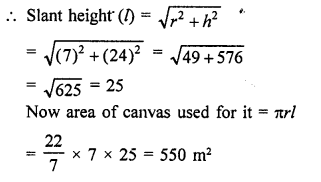Question 7 : Find the area of metal sheet required in making a closed hollow cone of base radius 7 cm and height 24 cm. making a closed hollow cone of base radius 7 cm and height 24 cm.

Base radius of the closed cone (r) = 7 cm
and vertical height (h) = 24 cm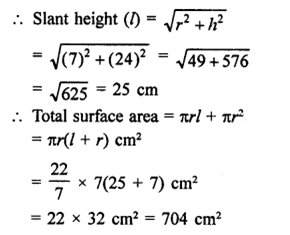Question 8 : Find the length of cloth used in making a conical pandal of height 100 m and base radius 240 m, if the cloth is 100π m wide.

Height of conical pandal (A) = 100 m
Base radius (r) = 240 m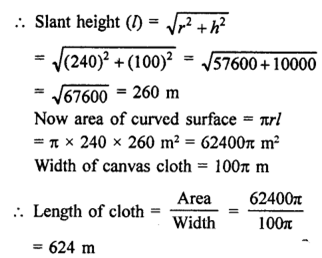Todays Deals### RD Chapter 20- Surface Areas and Volume of A Right Circular Cone Ex-VSAQS Contributorskrishan

Name:
Email:

# Latest News# 9000 interview questions in different categories